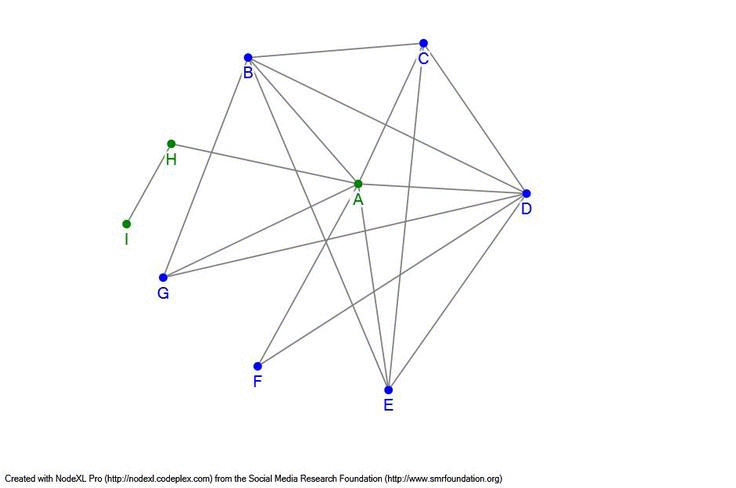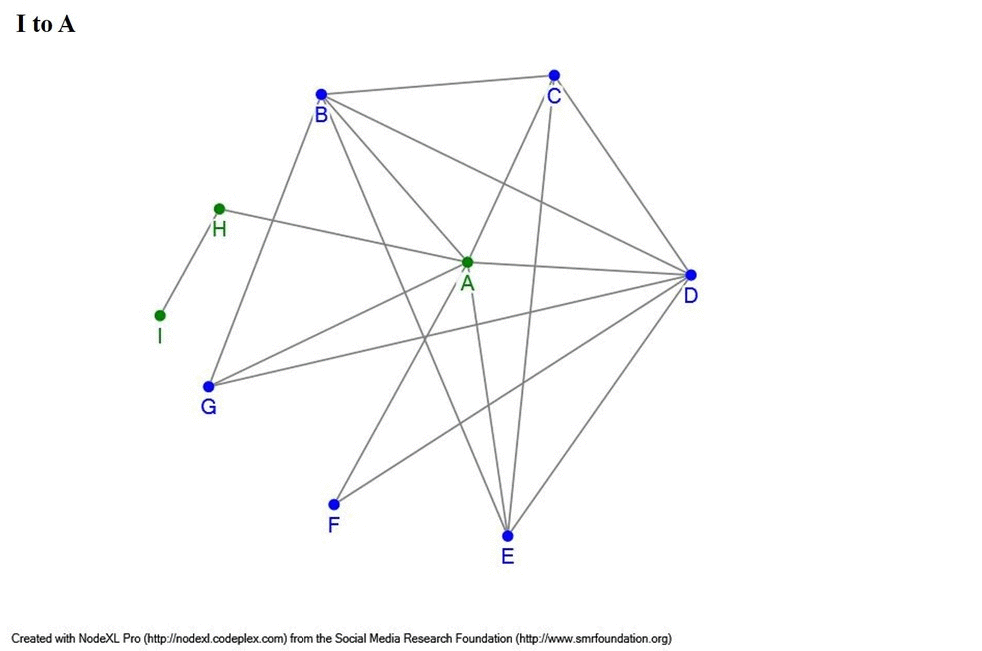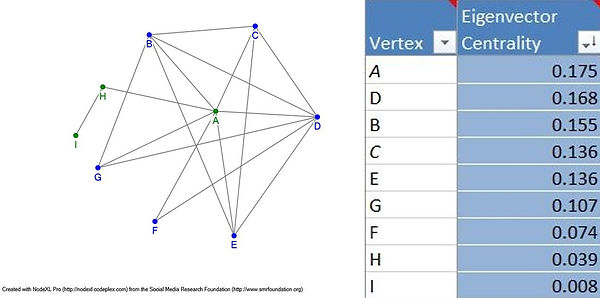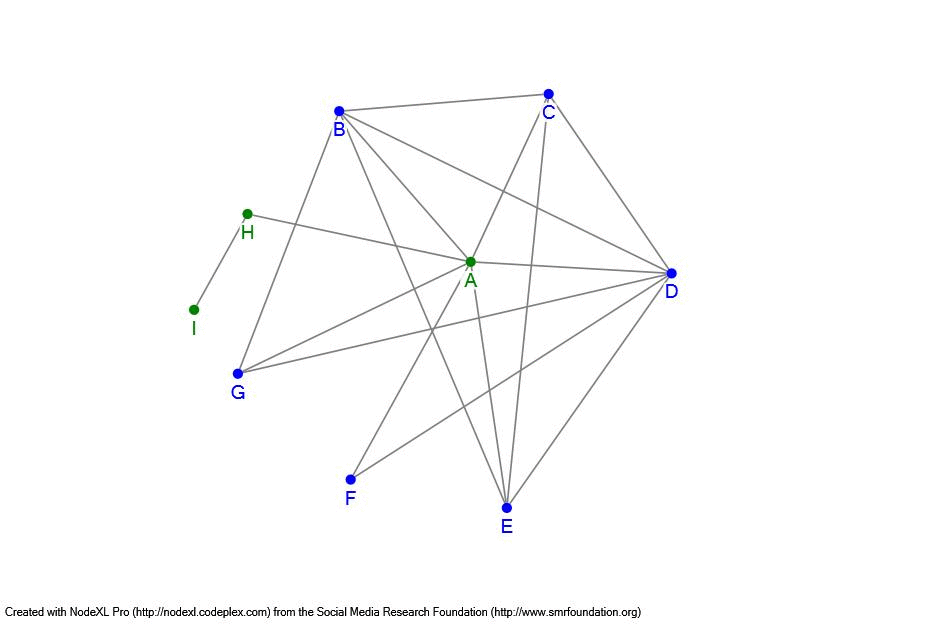top of page## What is social network analysis?

This project is a digital humanities thesis.  Digital humanities simply means the study of a humanities subject with the application of technology to quantify it.

Social Network analysis is a way to study relationships between people.  It provides visual and statistical data to explain a social situation through graphs made of nodes and edges.  All the graphs created for this project were made with NodeXL.  The network here shows a fictional graph of 9 nodes and 17 edges to explain the basics of this method.  Refer back to this page for definitions of network terms used to describe the Salem Witch Trials networks featured on this site.Nodes:

A node represents a person.   On the example,  the labelled dots are all nodes.  In the Salem networks, labels are removed for clarity, but the dots represent individuals.  If a graph includes 9 nodes, then that means it includes 9 people.  The gif below shows the diagram with the nodes and their edges highlighted.Edges:

The lines extended from each node are edges.  Each edge represents a relationship between nodes.  On the example, nodes A and B are connected with an edge, which means A and B have a relationship.  Each network can have different standards for relationships, so in each section the definition is clearly stated to explain what counts and does not count as a relationship.  The example below shows each of the 17 edges in this network.Edge Weight:

The edge weight refers to the frequency of which an edge appears in the data set.  When the network is graphed, it merges repeated edges into one to keep the graph relatively neat.  The AB edge may appear more often than the AC edge, so it is worth considering the relevance of this information.  Note that the edge weight is based solely on documents, so other factors (kinship, number of documents, etc.) may contribute to the interpretation of this data.Degree:

A degree is the number of edges or connections extending from a node.  The more degrees a node has, the better connected the node is within a network.  If you look below at the example graph with the degree data, you can see how A is clearly the most connected and I is the least.Geodesic Distance:

The shortest path between different nodes is the geodesic distance.  The distance between A and I is 2 since you need to travel from I to H and then H to A.  In comparison, B has a distance of 3 from I since it must travel the same route as A, but also B to A.  The gif below illustrates this difference.  Travel to most other nodes is much shorter given the closeness of the graph since most nodes are connected through A.  The average geodesic distance is 1.5, and the maximum distance is 3.  Note that this is the shortest distance, so node E could travel to D through B, C, and then D, but the distance is calculated by the shortest path.Betweenness Centrality:

The Betweenness Centrality is based on the shortest distance between nodes.  It calculates how frequently a node is a part of the shortest distance to another node.  If node F wants to reach node B, both A and D are the shortest path since both allow F to reach B with a distance of 2.  The shortest path to node I must always include H, so H is involved in the 7 paths for nodes A through G to reach I.  Node I is never the shortest path to another node given its isolation in the network.  The data set below shows the Betweenness Centrality for the example graph.  Notice how aside from I, nodes C, E, G, and F also receive a score of zero.  These nodes connect to several nodes on the network, but never create the shortest path.  A is clearly the easiest node to travel elsewhere in the network.  Node A's position surpasses C, E, G, and F's connections since it creates shorter pathways through the network.Eigenvector Centrality:

The Eigenvector Centrality ranks nodes based on how important or influential their position within the network is in comparison to the other nodes.  It depends on the node and the nodes it is connected to in the network.  Node A is the most connected node in this network example, but its connections are also well connected in the network.  This allows A to receive the highest Eigenvector Centrality.  However, if you compare nodes F and H, both are connected to two nodes.  H connects to A and I, and F connects to A and D.  If the Eigenvector score only depended on the connections, F and H are equal, but F's score is nearly twice as high as H's score.  Both connect to node A, so they are equal in that regard, but nodes D and I are quite different.  Node D is the second most connected node in the network and I is the least.  F is therefore connected to a much better node to know in comparison to H.  F's relationship with D raises its Eigenvector score much more than H's relationship with I.  It matters who you know, and who your connections know to receive a high Eigenvector score.Groups:

In the provided network, nodes A, H, and I are green.  Nodes B, C, D, E, F, and G are blue.  These colors represent the sorted groups of the network.  These groups can be manipulated by node attributes or grouped by NodeXL's algorithm to group by cluster.  In this case, the groups are based on clusters.  These clusters represent subgroups within a network.  When grouping with NodeXL's algorithms, it poses the challenge of determining why a calculated group has its own community within the network.  It opens the door for some new discoveries about networks by grouping people previously not closely linked through tradition study.bottom of page